# 1Ph Fault Analysis with PIC16F73 with GSM

In this project we will learn how to identify faults in single phase line and additionally we will send the fault notification to a mobile number as SMS. In this project we will use PIC16F73 micro-controller and SIM module as GSM module. We’ll also know why we need single phase fault protection and how we can do this.

Disclaimer: Electricity is always dangerous. Skill required to work with electricity. Do work with your own risk. Author will not be responsible for any misuse or harmful act. This website contents is also copyright protected. Anything copied and directly posted in your website and claiming it as yours is prohibited and nonsensical. Author published all the articles as opensource to help you making your project and learning purpose only. Learn and make one for yourself. If need any help feel free to ask the author. Author will be helpful to you. Thanks.

Table of Contents

## Faults in single phase line:

The main faults in single phase line is line voltage. Either the line voltage goes too high or too low is not suitable for our home appliances. Most of us have power line rated of 220V but in some countries it may be different. Whatever it is, too high or too low voltage is not good for appliances. Also spikes in power line is not acceptable. A single spike may damage our appliance. That is why we must pay attention in line voltage to provide protection.

Another protection we can keep is high current protection. Most of the houses have circuit breakers and fuses. These two device can give short circuit protection. Besides, we can add high current protection. When a load or a set of load is taking extra current than it’s rated current, we can trip that load for a while. If this same case happen again and again, we can trip permanently.

## Voltage and Current Sensing:

### Voltage sensing:

To sense line voltage and current, we need some kind of device which can convert or transform the ratio. Most of the control circuits works around 5V range. So if we want to sense some kind of parameters, we have to convert that parameter into voltage range of 5V. Here in our project, we need to sense the line voltage and Load current. As line voltage is 220V AC, we can not sense this range directly with our sensing device (here PIC16F73 micro-controller). So we will use a transformer, formally known as potential transformer.A simple Transformer

A simple transformer with ratio 220:12 can be used as our potential transformer. Then this transformer will convert the 220V into 12V range. And one more step will make it suitable for our micro-controller.

### Current Sensing:

Similarly to sense current we need another type of transformer called Current Transformer (CT) which converts high rated current into small rated current. To know more about current transformer please check this.Current Transformer

As we can not sense any current rather than voltage, so we need to convert the secondary small current into voltage bypassing that current through a burden resistor.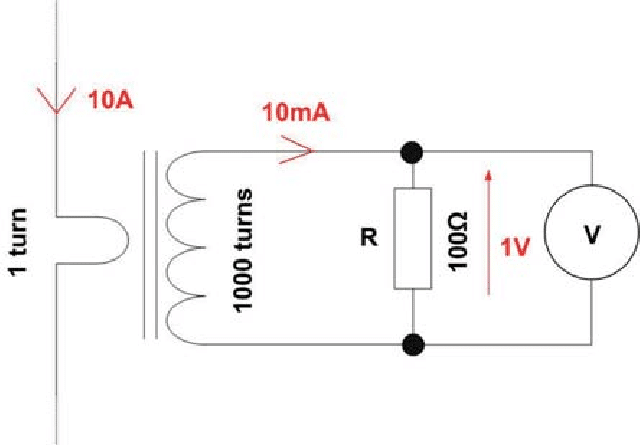Burden resistor with CT

Calculation is very simple, V=IR. Using this simple equation, we can easily find the line current.

## PIC16F73 micro-controller:

PIC16F73 is a very common 8bit micro-controller with 28pin package with 22 I/O. 6 channel 8bit ADC (AN0-AN5) makes it easy to sense voltages. A general feature can be found in this image: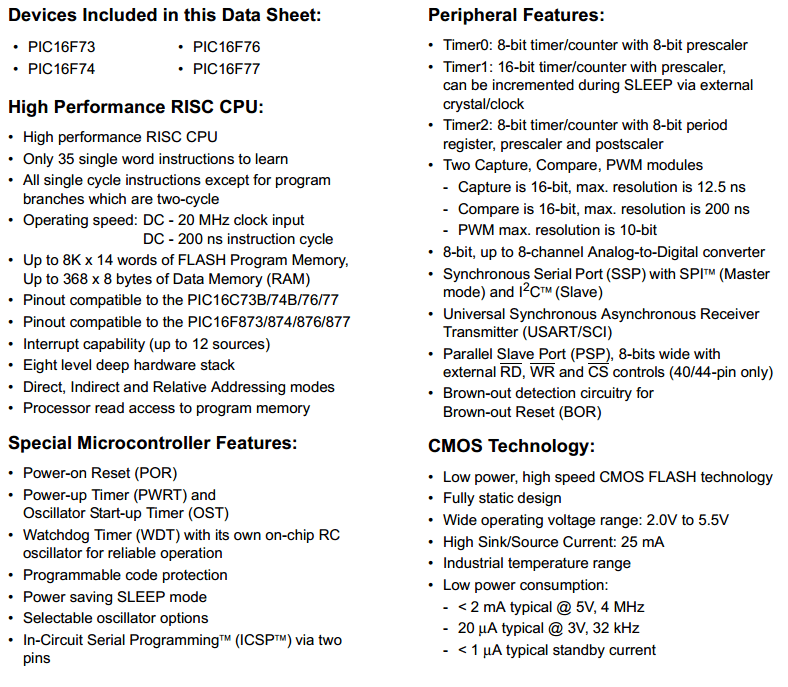PIC16F73 basic feature page

Each MCU have various special features. We need to use whatever we need for a purpose. Here in this project, we need ADC feature for sensing voltage and current. We will also need another feature later which we’ll discuss later on.

### Analog to Digital Converter:

Analog to Digital Converter is a kind of converter which converts analog voltage into digital data. You can find more about ADC here. PIC16F73’s ADC features are found here.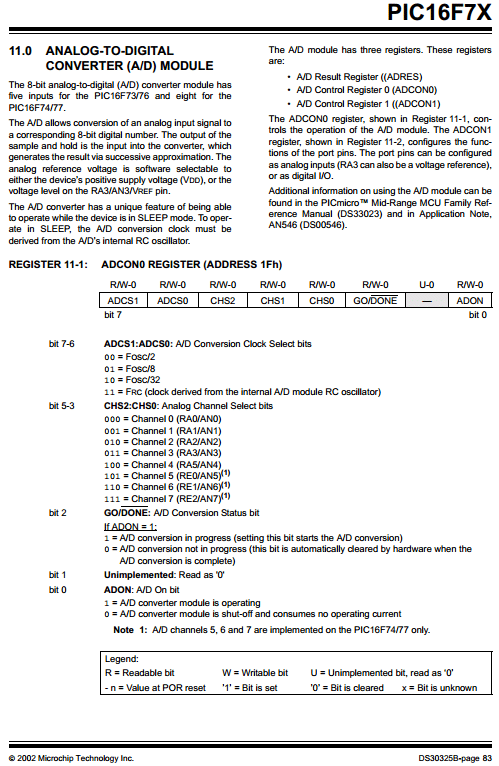PIC16F73 ADC settings 1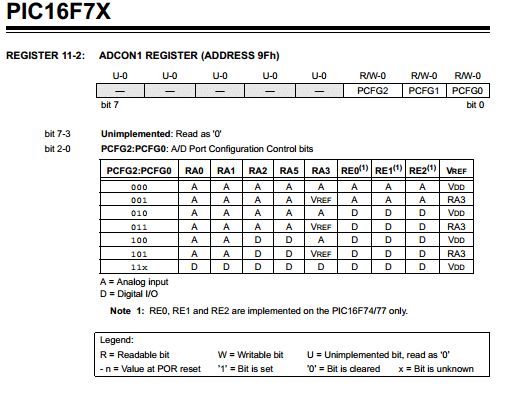PIC16F73 ADC settings 2

Here we have some kind of registers to command the ADC for a specific process. This will be discussed later.

This far we have seen the sensing techniques. After taking the input readings we can process our data and take decision what to do next.

## Load Control Device:

To turn the load on/off keeping the control device isolated we can use Relays.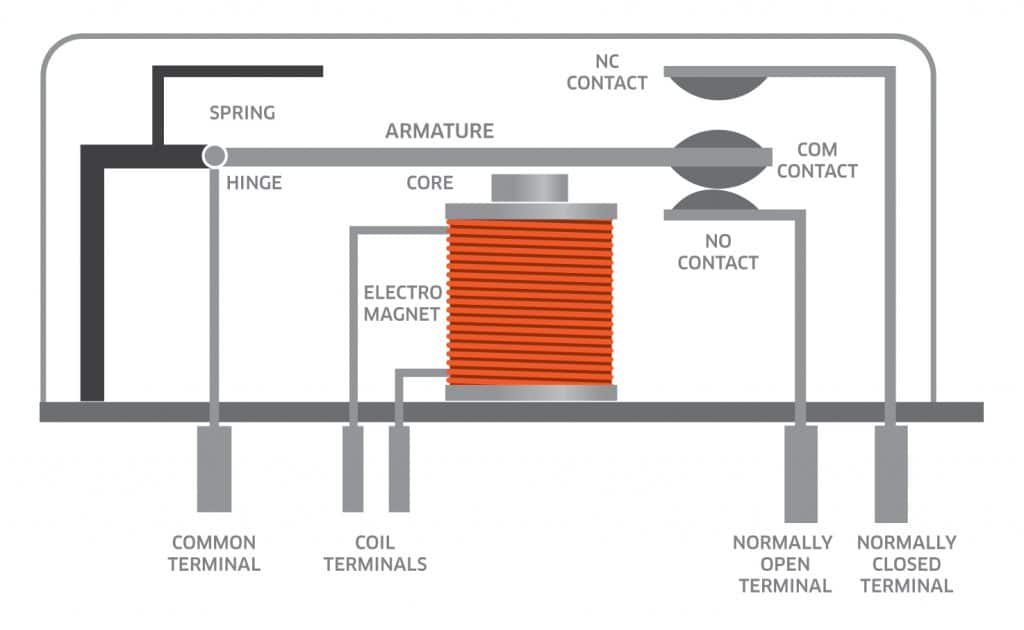Inside mechanism of a relay

Relay can provide isolated switching and easy to use too. So for our project we’ll use relay as the elector-mechanical switch.

## Communicating (GSM) Device:

Another part of our project is to notify the fault condition via SMS. For this purpose, we will use a GSM module. Any GSM module can be used, here we used SIM300 based GSM module. You can use any GSM module.Sim300 based GSM module and SIM800L GSM module

GSM module works through UART communication protocol. And supports ‘AT’ commands. You can read SIM300 AT command set here and SIM800L AT Command set here. Both module have similar AT command set to communicate with them. The simple communication technique between micro-controller and GSM module is: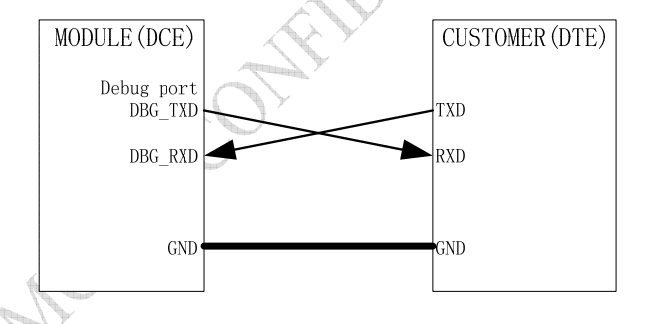GSM module to MCU connection diagram via UART protocol

The communication(UART) works at multiple baud rate but most of the cases the default baud rate is 9600. So you can set your baud rate at 9600. Another key point is, the SIM module have lower logic level (3.3V). On the other hand MCU have logic level of 5V. This may create problem to GSM module’s Rx pin. That is why you will need to use a voltage divider like this: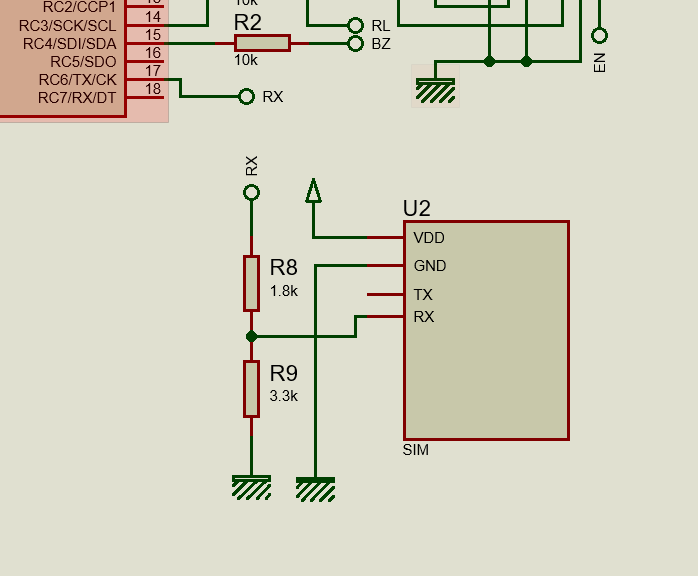Simple logic level matching using resistor voltage divider

When the MCU transmitting at 5V logic level, this voltage divider will lower the voltage to 3.3V level. As we are not receiving any SMS from GSM module in this project so we do not need to use the Tx pin of GSM module. But if we need to use it, we can use this connection directly. Because, MCU can accept logic level from 2.5V. Anyway, for this project we will not need any further connection so don’t worry.

## Block Diagram:

Here is our project block diagram. The main input line (AC220V) which we will monitor with a CT and PT then will be switched via relay to load. The micro-controller get reading of current and voltage from CT and PT correspondingly and decide when to turn the relay on/off with the help of a relay driver. Information displayed on LCD which is a common 16X2 LCD display. A GSM module is here for sending SMS. And finally we need a power supply to power the devices up. For microcontroller we need 5V but for relay we need 12V.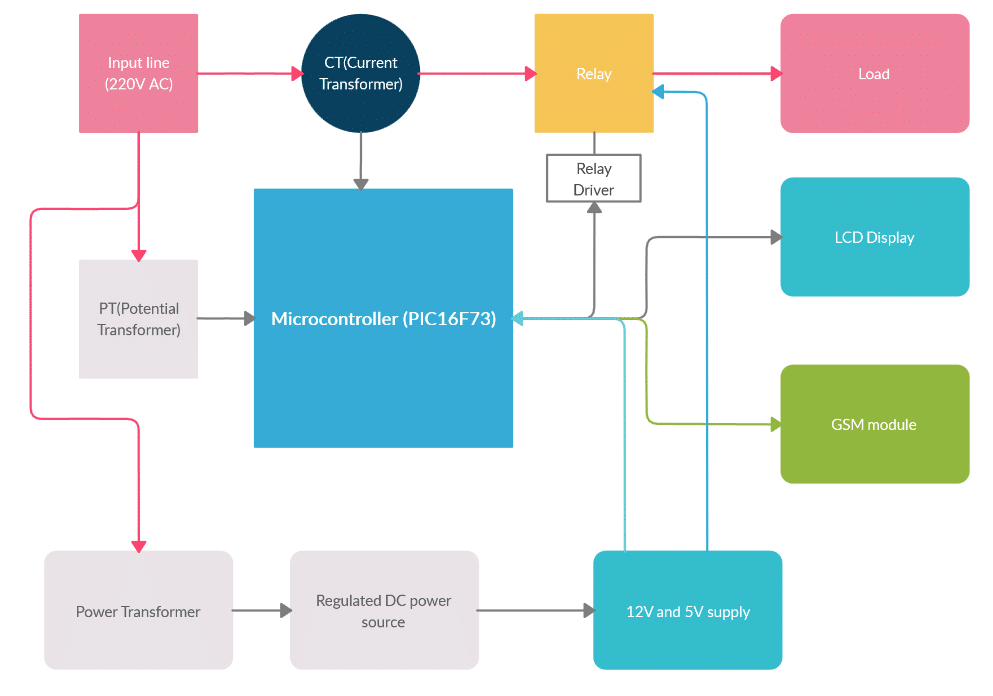Block diagram

## Circuit diagram:

Here is the compete circuit diagram of our project.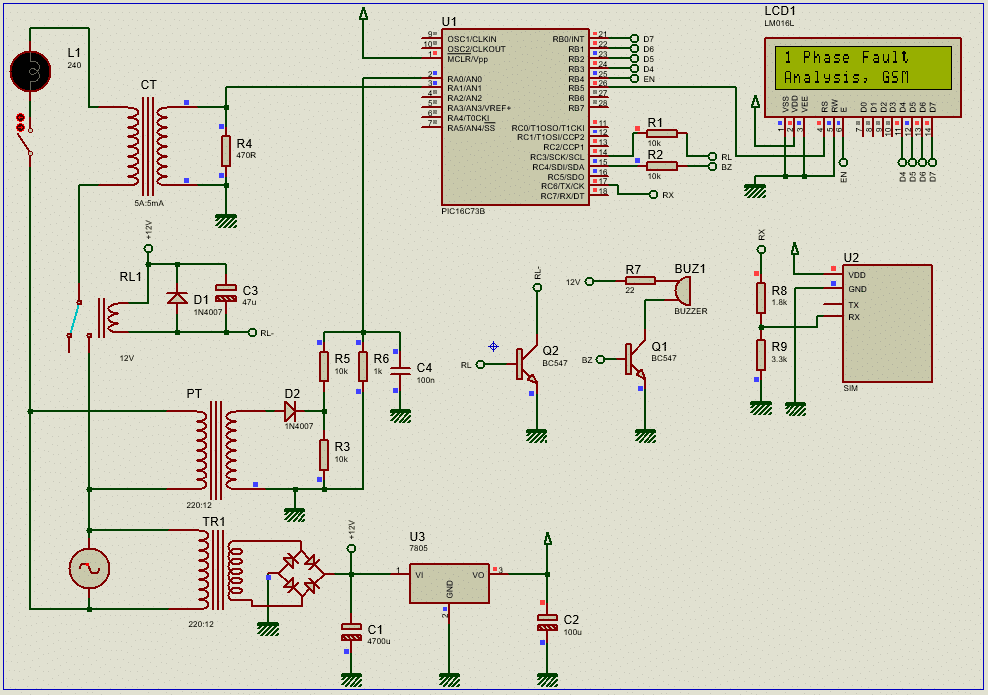Circuit diagram of the project

Here you can see different components including transformers, LCD, micro-controller and other associated devices.

## Connection summery:

As mentioned earlier to sense line voltage and load current we’ll need two transformers named PT and CT. Starting from the source, we have TR1 as power transformer which is providing 12V AC and after that a bridge diode and a capacitor(C1) is making it unregulated DC12V. This 12V(UG) is supplied to relay and the voltage regulator IC(U3) LM7805CV. A capacitor C2 is added just after the voltage regulator to make it more smooth. The regulated 5V DC supply is supplied to micro-controller(PIC16F73) and LCD display. The GSM module is supplied separately in this project.

Now, PT (220:12) is connected parallel to the supply and CT and relay(RL1) are connected in series with the line and the load(L1) via a switch. [Note: In practice, we’ve to use several lamps and switches]. Diode D1 and capacitor C3 is connected with the relay coil which is driven by a transistor Q2(BC547) which is our relay driver in this project. D1 used to remove the back emf generated by the relay coil and the capacitor C3 stabilize the coil magnetic flux.

After PT, diode D2 rectify the voltage and resistor R3 is used as a shunt resistor to eliminate noise and helps flowing minimum current through the diode D2. Then this voltage is divided with the help of R5 and R6 voltage divider resistor. A ceramic capacitor C4 is used here to remove noise. This voltage is then supplied to RA0(AN0) of the micro-controller.

R4 is working as the burden resistor of our CT which is converting the current into voltage from CT secondary winding. This voltage is then supplied to RA1(AN1) of the micro-controller.

Using ADC module of MCU line voltage and current is then calculated.

The Display (LCD) need 4 data pins and 2 control pins to work properly which are connected to the MCU (RB0 to RB5). A resistor (usually 2.7K) need to use in real hardware in between VEE and GND to set the LCD contrast.

GSM module(U2) is connected in common GND line and the Tx pin of MCU is connected to the Rx pin of GSM module through two resistors ( logic level reducer) R8 and R9. The Tx pin is the pin of UART module of our micro-controller.

And finally we will add a buzzer as an audio alarming system which is driven with the help of a transistor Q1. Both of the transistors (Q2 and Q1) are biased through 10K resistors.

The Vpp/MCLR pin of the micro-controller is connected to 5V supply ( through a 10K resistor in hardware). And a 8MHz crystal is used in real hardware which is not shown in circuit diagram.

## Flow chart: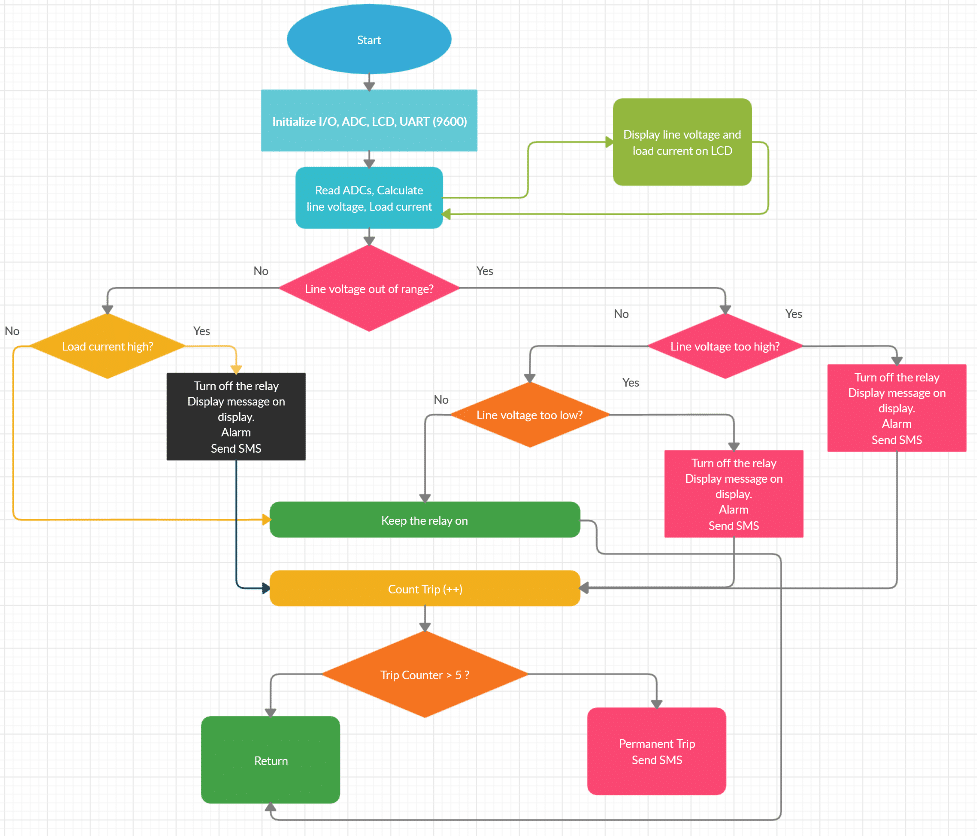Flow Chart

## mikroC Code:

```/*******************************************************************************
*          Program for, "1Ph fault analysis and senging sms through GSM"       *
*                Program Written by_ Engr. Mithun K. Das                       *
*                      MCU:PIC16F73B; X-Tal: 8MHz                              *
*                         Date:27-08-2016                                      *
*******************************************************************************/
// LCD module connections
sbit LCD_RS at RB5_bit;
sbit LCD_EN at RB4_bit;
sbit LCD_D4 at RB3_bit;
sbit LCD_D5 at RB2_bit;
sbit LCD_D6 at RB1_bit;
sbit LCD_D7 at RB0_bit;

sbit LCD_RS_Direction at TRISB5_bit;
sbit LCD_EN_Direction at TRISB4_bit;
sbit LCD_D4_Direction at TRISB3_bit;
sbit LCD_D5_Direction at TRISB2_bit;
sbit LCD_D6_Direction at TRISB1_bit;
sbit LCD_D7_Direction at TRISB0_bit;
// End LCD module connections
void Lcd_COut(char row, char col, const char *cptr)
{
char chr = 0;             //first, it is used as empty string
Lcd_Out(row, col, &chr);  //nothing to write but set position.
for ( ; chr = *cptr ; ++cptr ) Lcd_Chr_CP(chr); //out in loop
}
void UART_Write_CText(const char *cptr)
{
char chr;
for ( ; chr = *cptr ; ++cptr ) UART1_Write(chr);
}
unsigned int voltage=0,current=0;
char volt[]="V:000V ";
void Get_Voltage(void)
{
unsigned int temp0=0;
unsigned int maxpoint0=0;
unsigned int k=0;

ADCON0 = 0b00000001;// AN0 selected
for(k=0;k<1000;k++)
{
if(temp0 = ADC_Read(0),temp0>maxpoint0)
{
maxpoint0 = temp0;
}
}
maxpoint0 = abs(ceil(maxpoint0));
voltage = maxpoint0*3.477;// (5/255)*11.00*0.707*220/9*cal_factor;
volt = (voltage/100) +48;
volt = (voltage/10)%10 +48;
volt =  voltage%10 +48;
Lcd_Out(1,1, volt);
Delay_ms(20);
maxpoint0 = 0;
}
char curr[]="I:0.00A";
void Get_Current(void)
{
//char message4[] = "0.000A";
unsigned int temp1=0;
unsigned int maxpoint1=0;
int kk=0;

ADCON0 = 0b00000101;// AN1 selected
for(kk=0;kk<3000;kk++)
{
if(temp1 = ADC_Read(1),temp1>maxpoint1)
{
maxpoint1 = temp1;
}
}
current = maxpoint1*0.340;// get the load current
curr = current/100+48;
curr = '.';
curr = (current/10)%10 +48;
curr = (current/1)%10 +48;
Lcd_Out(1,9,curr);
Delay_ms(20);
maxpoint1 = 0;
}
void Send_SMS_Int(void)
{
UART1_Write_Text("AT\r\n");
Delay_ms(200);
UART1_Write_Text("AT+cmgf=1\r\n");
Delay_ms(200);
UART1_Write_Text("AT+cmgs=");
UART1_Write((char)'"');
UART1_Write_Text("xxxxxxx");  //add your mobile number here
UART1_Write((char)'"');
UART1_Write((char)13);
UART1_Write((char)10);
Delay_ms(2000);
}
#define     Relay     RC4_bit
#define     Buzzer    RC5_bit
unsigned int cnt_ol=0,trip_cnt=0;
bit permanent_trip,sms_cnt;
void main()
{
TRISA = 0xFF;//all input
TRISC = 0x00;//all output
ADCON1 = 0x00;//all analog in
Relay = 0;
Buzzer = 1;
Lcd_Init();//int LCD
Lcd_Cmd(_LCD_CLEAR);
Lcd_Cmd(_LCD_CURSOR_OFF);
Lcd_COut(1,1,"1 Phase Fault");
Lcd_COut(2,1,"Analysis, GSM");
UART1_Init(9600);
Delay_ms(50); Buzzer = 0;
Delay_ms(2000);
Lcd_Cmd(_LCD_CLEAR);
UART_Write_CText("AT\r\n");Delay_ms(100);
UART_Write_CText("AT\r\n");Delay_ms(100);
UART_Write_CText("AT\r\n");Delay_ms(100);//send AT command
permanent_trip=0;
sms_cnt=1;
while(1)
{
OERR_bit = 0;// clear Overrun Error bit
FERR_bit = 0;//clear Farming Error bit
UART_Write_CText("AT\r\n");
if(permanent_trip==0)
{
Get_Voltage(void);
Get_Current(void);
if(voltage>180 && voltage<240)// voltage is in between
{
if(current<75)//not overloaded
{
Relay = 1;
Lcd_COut(2,1,"Normal Condition");
Delay_ms(50);
sms_cnt = 1;//reset mask
}
else
{
cnt_ol++;
if(cnt_ol>5)
{
Relay = 0;//trip
trip_cnt++;
cnt_ol = 0;//clear cnt
Lcd_COut(2,1,"Tripped,Overload");
Buzzer = 1;
Delay_ms(2000);
Lcd_Cmd(_LCD_CLEAR);
Buzzer = 0;
Lcd_COut(1,1,"Sending SMS_");
Send_SMS_Int(void);
UART_Write_CText("System is Tripped due to overload.");
Delay_ms(300);
UART1_Write((char)26);
Delay_ms(300);
Lcd_COut(2,1,"SMS Sent_");
Delay_ms(1000);
Lcd_Cmd(_LCD_CLEAR);
cnt_ol = 0;
}
}
}
else
{
Relay = 0;
if(voltage<=180)Lcd_COut(2,1,"Low Voltage");
if(voltage>=240)Lcd_COut(2,1,"High Voltage");
Delay_ms(1000);
trip_cnt++;
Lcd_COut(2,1,"Tripped, V_Error");
Buzzer = 1;
Delay_ms(2000);
Buzzer = 0;
Lcd_Cmd(_LCD_CLEAR);
Lcd_COut(1,1,"Sending SMS_");
Send_SMS_Int(void);
UART_Write_CText("System is Tripped due to Voltage error.");
Delay_ms(300);
UART1_Write((char)26);
Delay_ms(300);
Lcd_COut(2,1,"SMS Sent_");
Delay_ms(1000);
Lcd_Cmd(_LCD_CLEAR);
}
}
else
{
Lcd_Cmd(_LCD_CLEAR);
Lcd_COut(1,1,"Permanently");
Lcd_COut(2,1,"Tripped");
Delay_ms(3000);
if(sms_cnt)
{
Lcd_Cmd(_LCD_CLEAR);
Lcd_COut(1,1,"Sending SMS_");
Send_SMS_Int(void);
Buzzer = 1;
UART_Write_CText("System is Permanently Tripped.");
Delay_ms(300);
UART1_Write((char)26);
Delay_ms(300);
sms_cnt = 0;
Lcd_COut(2,1,"SMS Sent_");
Delay_ms(1000);
Buzzer = 0;
Lcd_Cmd(_LCD_CLEAR);
}
}

if(trip_cnt>5)
{
permanent_trip = 1;
}
else
{
permanent_trip = 0;
}

}//while(1)
}//void main```

Download mikroC project file from here.

## Code Explanation:

Code is easy and written in simple way so that you can understand easily.

```// LCD module connections
sbit LCD_RS at RB5_bit;
sbit LCD_EN at RB4_bit;
sbit LCD_D4 at RB3_bit;
sbit LCD_D5 at RB2_bit;
sbit LCD_D6 at RB1_bit;
sbit LCD_D7 at RB0_bit;

sbit LCD_RS_Direction at TRISB5_bit;
sbit LCD_EN_Direction at TRISB4_bit;
sbit LCD_D4_Direction at TRISB3_bit;
sbit LCD_D5_Direction at TRISB2_bit;
sbit LCD_D6_Direction at TRISB1_bit;
sbit LCD_D7_Direction at TRISB0_bit;
// End LCD module connections```

This part of the code is to setup the LCD connection with the micro-controller. Rest of the process is done by mikroC LCD library.

```void Lcd_COut(char row, char col, const char *cptr)
{
char chr = 0;             //first, it is used as empty string
Lcd_Out(row, col, &chr);  //nothing to write but set position.
for ( ; chr = *cptr ; ++cptr ) Lcd_Chr_CP(chr); //out in loop
}```

This sub-function is used to reduce RAM consumption for LCD functions. This way we can add more texts in LCD function.

```void UART_Write_CText(const char *cptr)
{
char chr;
for ( ; chr = *cptr ; ++cptr ) UART1_Write(chr);
}```

This part is also reducing RAM consumption when using UART function. It also helps adding more texts in UART function.

```unsigned int voltage=0,current=0;
char volt[]="V:000V ";
void Get_Voltage(void)
{
unsigned int temp0=0;
unsigned int maxpoint0=0;
unsigned int k=0;

ADCON0 = 0b00000001;// AN0 selected
for(k=0;k<1000;k++)
{
if(temp0 = ADC_Read(0),temp0>maxpoint0)
{
maxpoint0 = temp0;
}
}
maxpoint0 = abs(ceil(maxpoint0));
voltage = maxpoint0*3.477;// (5/255)*11.00*0.707*220/9*cal_factor;
volt = (voltage/100) +48;
volt = (voltage/10)%10 +48;
volt =  voltage%10 +48;
Lcd_Out(1,1, volt);
Delay_ms(20);
maxpoint0 = 0;
}```

In this sub-function we are calculating the line voltage. As we are taking reading of the AC signal directly so we need to find the peak point of each pulse. We are taking 1000 samples and comparing if it is higher than previous one. Once we find the previous one is greater or equal than the recent one, then that previous one will be the peak reading.

You can read AC voltage measurement technique to know more about this.

From that peak reading, we can easily calculate the line voltage taking the rms voltage equation in our calculation.

In this sub-function we are displaying the reading on our LCD. As LCD works in ASCII so we have to convert the decimal value into ASCII value.

```char curr[]="I:0.00A";
void Get_Current(void)
{
//char message4[] = "0.000A";
unsigned int temp1=0;
unsigned int maxpoint1=0;
int kk=0;

ADCON0 = 0b00000101;// AN1 selected
for(kk=0;kk<3000;kk++)
{
if(temp1 = ADC_Read(1),temp1>maxpoint1)
{
maxpoint1 = temp1;
}
}
current = maxpoint1*0.340;// get the load current
curr = current/100+48;
curr = '.';
curr = (current/10)%10 +48;
curr = (current/1)%10 +48;
Lcd_Out(1,9,curr);
Delay_ms(20);
maxpoint1 = 0;
}```

In this sun-function, we are calculating the load current. This one is using similar technique like the voltage reading.

```void Send_SMS_Int(void)
{
UART1_Write_Text("AT\r\n");
Delay_ms(200);
UART1_Write_Text("AT+cmgf=1\r\n");
Delay_ms(200);
UART1_Write_Text("AT+cmgs=");
UART1_Write((char)'"');
UART1_Write_Text("xxxxxxx");  //add your mobile number here
UART1_Write((char)'"');
UART1_Write((char)13);
UART1_Write((char)10);
Delay_ms(2000);
}```

In this part, we have kept a sub-function to send SMS. It initialize the GSM module to send a text as SMS to the given number. Whenever we need to send a SMS, we can call this function then print our text and send the end command.

```#define     Relay     RC4_bit
#define     Buzzer    RC5_bit
unsigned int cnt_ol=0,trip_cnt=0;
bit permanent_trip,sms_cnt;```

This is for simple pin declaration and some variable declaration.

``` TRISA = 0xFF;//all input
TRISC = 0x00;//all output
ADCON1 = 0x00;//all analog in
Relay = 0;
Buzzer = 1;
Lcd_Init();//int LCD
Lcd_Cmd(_LCD_CLEAR);
Lcd_Cmd(_LCD_CURSOR_OFF);
Lcd_COut(1,1,"1 Phase Fault");
Lcd_COut(2,1,"Analysis, GSM");
UART1_Init(9600);
Delay_ms(50); Buzzer = 0;
Delay_ms(2000);
Lcd_Cmd(_LCD_CLEAR);
UART_Write_CText("AT\r\n");Delay_ms(100);
UART_Write_CText("AT\r\n");Delay_ms(100);
UART_Write_CText("AT\r\n");Delay_ms(100);//send AT command
permanent_trip=0;
sms_cnt=1;```

This is for initialization of input/outputs, ADC settings, LCD initialization, UART initialization etc. Here we named our project on LCD and sent 3 ‘AT’ command to our GSM module to establish UART communication.

```    OERR_bit = 0;// clear Overrun Error bit
FERR_bit = 0;//clear Farming Error bit
UART_Write_CText("AT\r\n");```

OERR and FERR are cleared and ‘AT’ command is sent periodically to maintain UART communication between the MCU and GSM module.

```if(permanent_trip==0)
{
Get_Voltage(void);
Get_Current(void);
if(voltage>180 && voltage<240)// voltage is in between
{
if(current<75)//not overloaded
{
Relay = 1;
Lcd_COut(2,1,"Normal Condition");
Delay_ms(50);
sms_cnt = 1;//reset mask
}
else
{
cnt_ol++;
if(cnt_ol>5)
{
Relay = 0;//trip
trip_cnt++;
cnt_ol = 0;//clear cnt
Lcd_COut(2,1,"Tripped,Overload");
Buzzer = 1;
Delay_ms(2000);
Lcd_Cmd(_LCD_CLEAR);
Buzzer = 0;
Lcd_COut(1,1,"Sending SMS_");
Send_SMS_Int(void);
UART_Write_CText("System is Tripped due to overload.");
Delay_ms(300);
UART1_Write((char)26);
Delay_ms(300);
Lcd_COut(2,1,"SMS Sent_");
Delay_ms(1000);
Lcd_Cmd(_LCD_CLEAR);
cnt_ol = 0;
}
}
}
else
{
Relay = 0;
if(voltage<=180)Lcd_COut(2,1,"Low Voltage");
if(voltage>=240)Lcd_COut(2,1,"High Voltage");
Delay_ms(1000);
trip_cnt++;
Lcd_COut(2,1,"Tripped, V_Error");
Buzzer = 1;
Delay_ms(2000);
Buzzer = 0;
Lcd_Cmd(_LCD_CLEAR);
Lcd_COut(1,1,"Sending SMS_");
Send_SMS_Int(void);
UART_Write_CText("System is Tripped due to Voltage error.");
Delay_ms(300);
UART1_Write((char)26);
Delay_ms(300);
Lcd_COut(2,1,"SMS Sent_");
Delay_ms(1000);
Lcd_Cmd(_LCD_CLEAR);
}
}
else
{
Lcd_Cmd(_LCD_CLEAR);
Lcd_COut(1,1,"Permanently");
Lcd_COut(2,1,"Tripped");
Delay_ms(3000);
if(sms_cnt)
{
Lcd_Cmd(_LCD_CLEAR);
Lcd_COut(1,1,"Sending SMS_");
Send_SMS_Int(void);
Buzzer = 1;
UART_Write_CText("System is Permanently Tripped.");
Delay_ms(300);
UART1_Write((char)26);
Delay_ms(300);
sms_cnt = 0;
Lcd_COut(2,1,"SMS Sent_");
Delay_ms(1000);
Buzzer = 0;
Lcd_Cmd(_LCD_CLEAR);
}
}

if(trip_cnt>5)
{
permanent_trip = 1;
}
else
{
permanent_trip = 0;
}```

And in this part inside the while(1) loop we are checking if the permanent _trip is over or not. If it is over then system is tripped permanently and if not system will check line voltage and current periodically.

Here we used a voltage range of 180 to 240 which is normal for a 220V line for common appliances. For sophisticated appliances you can narrow this range down as per your wish.

And current range is 75 is considering as 0.75A of current. Over that value system will trip as overload. You can set as per your requirement.

## PCB Design: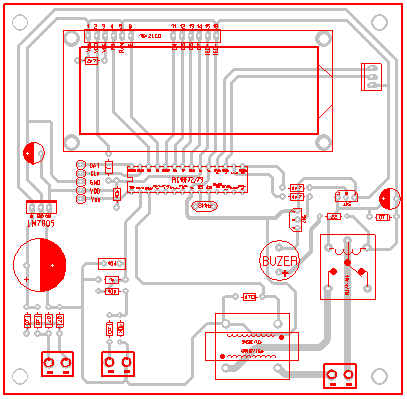PCB of our project

As this is an educational project so PCB is designed on that manner. For commercial use, this system will be designed in commercial way.

#### MKDas

Mithun K. Das; B.Sc. in EEE from KUET. Blog: https://labprojectsbd.com

### 1 Comment#### enrique · September 12, 2020 at 3:41 pm

Excellent all your work, I am a faithful follower from Colombia, thank you very much for sharing your knowledge, I wish you many successes, I look forward to your next work